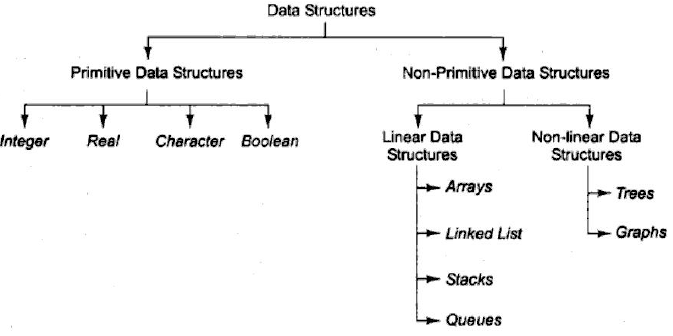# Introduction to Data Structures

In this article we will have Introduction to Data Structures. Data is set of values. A single unit of value is called data item. Collection of data is usually organised into fields, records and files.

Data can be organised in different ways. The logical and mathematical model of a particular organization of data is called data structure. Data structures are generally classified into two types:

• Primitive structures: For example integer, real, character, Boolean. These data types are simple as these cannot be further sub-divided.
• Non-primitive structures: For example: Arrays, Trees, Linked List etc. These are complex data types. Based on the arrangement of data, the primitive structures can be further divided into two types: linear and non-linear data structure.
Linear data structure: In linear data structure, the data is arranged in linear fashion or sequential order. Some of the examples are arrays, linked lists, stacks, queues.
Non-linear data structure: In non-linear data structure, the data is not arranged in sequence. Some of the examples are trees, graphs.## Data Structure Operations

The data stored in data structure is accessed by means of operations. The choice of selecting data structure for particular situation depends upon the frequency operation. Some of the frequently used operations are:

• Insertion: Adding a new record in the data structure.
• Deletion: Remove a record from the data structure.
• Traverse: Accessing each record in the data structure exactly once.
• Search: Searching refers to the process of finding the location of record with given key value or finding the locations of all records which satisfy one or more condition.
• Sorting: Sorting means arranging the records in some pattern.
• Merging: Merging means combining the records of two different sorted files into a single sorted file.

## Abstract Data Type

Abstract Data Type (ADT) refers to set of data values and associated operations. ADT specifies the data type but is independent of its implementation details. Examples of ADT are stack, queues etc.

An algorithm is a well-defined sequence of steps used for solving certain sort of problems. The algorithm must be efficient in processing the data. The time and space are two major measures to judge the efficiency of an algorithm.

Complexity: The complexity of an algorithm is the function f(n) which determines the running time/space of algorithm in terms of input size ‘n’. It defines how fast or slow an algorithm is.

Time-Space Tradeoff: Time-space tradeoff means by increasing the amount of space required to store the data, the running time of algorithm decreases or by decreasing the amount of space required to store the data, the running time of algorithm increases.

There are generally three cases in complexity theory:

1. Worst case: the maximum value of f(n) for any possible input.
2. Average case: the expected value of f(n).
3. Best case: the minimum possible value of f(n).

## Big O Notation

The Big O notation defines an upper bound function g(n) for function f(n) which represents the time/space complexity of an algorithm for input of size n. This is all we need to need know about Big O notation from IBPS IT officer exam perspective.